# What is the goal of process capability

## Process capability indicators

This article explains the importance of process capability metrics Cp and Cpk. Cp stands for Capability of Processwhat translates Ability of the process means. The two key figures are dimensionless, i.e. no unit is assigned to them. The higher the metrics, the better the process. In the past, the minimum requirement was for Cp and Cpk≥ 1.33. This limit value is still common in many industries today. In other industries, such as the automotive industry, the minimum requirement is Cp and Cpk ≥1.66. But what do these two key figures say?

The aim of a production process is to always produce consistent quality features. For example, if we want to produce a screw, the critical quality features are fixed, such as screw length, thread pitch, yield point, etc.

### Example: length of a screw

The aim is to produce as many screws as possible, which ideally all have the same length (e.g. 15mm). This length is specified in the technical specification, with permissible deviations upwards and downwards. So there are maximum and minimum allowable dimensions for the screw length (tolerance). The screw produced has to be somewhere between the maximum and minimum dimensions, otherwise it is out of tolerance and thus scrap (reworking a single screw is in most cases not worthwhile). Let's assume the tolerance is ± 0.3mm.

If we now produce 1000 screws and measure every single screw, most screws will have a length of 15mm. But not all of them. Some screws will be longer than 15mm, others will be shorter than 15mm. The screws produced are normally distributed due to random influences in production.

### Normal distribution

To describe a normal distribution you need two parameters. The scatter and the mean. The mean value of the distribution is 15mm in our example. The standard deviation (of a sample) is denoted by the letter s and is a measure for determining the spread. Let us assume that the standard deviation s is 0.1 mm. Now we only need the required tolerance of ± 0.3mm to determine the capability indicators. The following figure shows the normal distribution of the characteristic screw length with the standard deviation and the tolerance limits.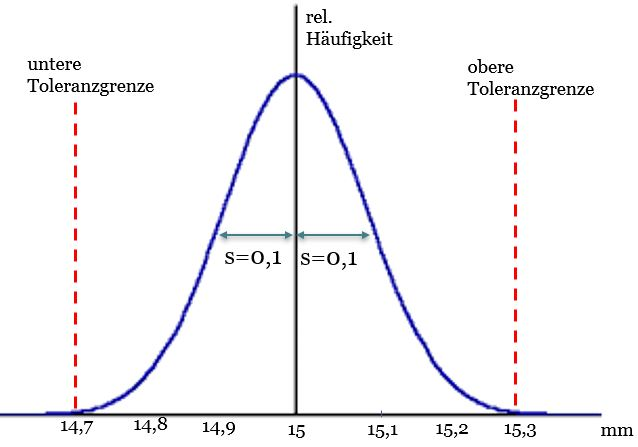### Determination of Cp

The meaning of Cp can be easily explained using the graphic above. Cp says how often six standard deviations s fit into the tolerance T of 6mm. The tolerance is the difference between the upper tolerance limit UGW and the lower tolerance limit UGW. Six standard deviations fit exactly 1x into the tolerance, since the tolerance is 0.6mm and the standard deviation is 0.1mm. The Cp value in this example is 1. How do we calculate this? Very easily...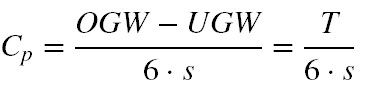So we simply divide the tolerance by six standard deviations and get the Cp value. Cp = 0.6mm / 0.6mm = 1.00.

Attention: Cp only provides information about the spreading behavior of our screw production. We have assumed for the example above that the mean value is exactly at our target value of 15mm. In this particular case, Cp = Cpk. But if the mean value is shifted from the target value of 15mm, then the maximum of our normal distribution approaches a tolerance limit.

### Determination of Cpk

It is irrelevant for the Cpk value whether our mean value for production deviates in the direction of OGW or UGW. The only important thing is how close the mean value comes to the tolerance limit. In the graphic below, the mean value is shifted by 0.1 mm in the direction of the upper tolerance limit. This means that most of the screws we produce are too long. Some are even so long that they are outside the upper tolerance. Cpk now describes how often 3 standard deviations fit into the distance between the upper tolerance limit and the mean value.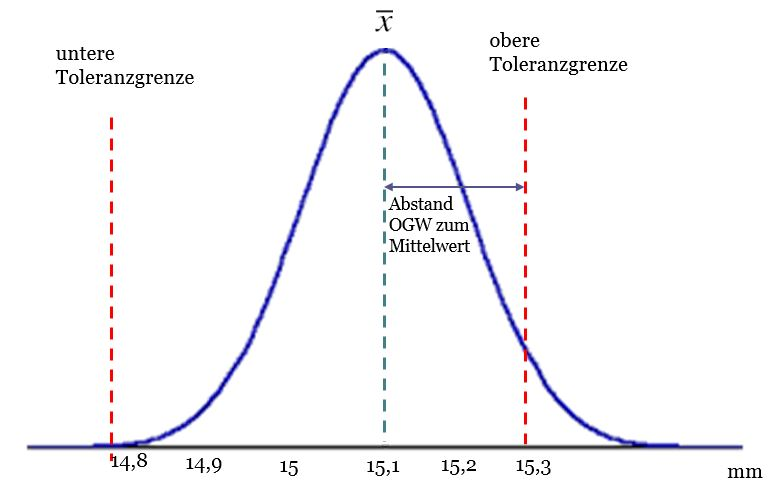If our screws were to approach the lower tolerance limit, then Cpk describes how often three standard deviations fit into the distance between the mean value and the lower tolerance limit. There are therefore two formulas for determining Cpk. These are referred to as Cpu (shift towards UGW) and Cpo (shift towards OGW).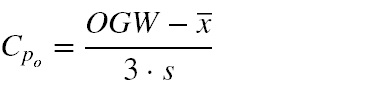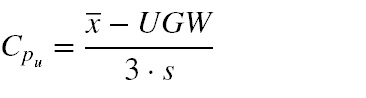For our example it results

Cpu = (15.1mm-14.7mm) / 0.3mm = 1.33

Cpo = (15.3mm - 15.1mm) / 0.3mm = 0.67

Since we are not interested in the better value (here Cpu), but rather the critical value (here Cpo), the worse value for Cpk is used. The result is Cpk = 0.67.

In our example, the process quality cannot be classified as capable, since both Cp and Cpk are <1.33.

In practice, the way to determine Cp and Cpk is somewhat more tedious. First, the short-term capacity Cm and Cmk is determined, which are calculated according to the same formulas, but under different framework conditions. The short-term indices Cm and Cmk are only replaced by Cp and Cpk when these preliminary key figures are stable over a long period of time.

Details
Written by Hartmut Winkler